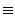Crypto Home

# Cancellation Property of Modular Arithmetic

## Property

Given a positive integer n and integers k, u, and v:

If k is relatively prime to n then

kukv (mod n)

may be simplified by canceling k from each side leaving.

uv (mod n)

## Proof

kukv (mod n)

ku - kv0 (mod n)

k*(u - v)0 (mod n)

k*(u - v) = j*n (for some integer j)

However, since the left hand side is a product of two integers one of which, k, is stated as being relatively prime to n. The consequence of this is that all of the factors of n must be contained in the integer represented by (u-v). Hence (u-v) must be divisible by n and therefore:

(u - v)0 (mod n)

uv (mod n)

## Important Note

Notice that the condition that k be relatively prime to n is critically relevant. For example:

6090 (mod 15)

Which can be written as

10*610*9 (mod 15)

However, the factor 10 cannot be cancelled from each side because 10 and 15 are not relatively prime. This underscores the fact that normal arithmetic division is an undefined operation in modular arithmetic. Don't do it. Ever. Even when it might appear obvious what the answer is. For instance, in the above example, if you want to "divide" both sides by 10, do so by multiplying both sides by the modulo-15 multiplicative inverse of 10. What you will discover in attempting to do so is that 10 has no multiplicative inverse in the modulo-15 world!

It is therefore important to remember that the property developed here is a cancellation property and not a division operation.